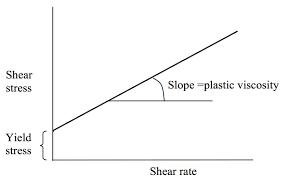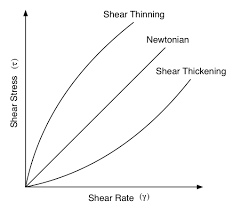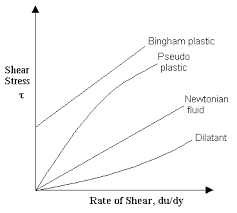## How to Calculate and Solve for Viscosity of Bingham Fluids | RheologyThe image above represents viscosity of bingham fluids.

To compute for viscosity of bingham fluids, three essential parameters are needed and these parameters are Yield Stress (ζy), Shear Rate (γ) and Co-efficient of Rigidity (ηB).

The formula for calculating viscosity of bingham fluids:

ηa = ηB + ζy / γ

Where:

ηa = Viscosity of Bingham Fluids
ζy = Yield Stress
γ = Shear Rate
ηB = Co-efficient of Rigidity

Let’s solve an example;
Find the viscosity of bingham fluids when the yield stress is 21, the shear rate is 18 and co-efficient of rigidity is 14.

This implies that;

ζy = Yield Stress = 21
γ = Shear Rate = 18
ηB = Co-efficient of Rigidity = 14

ηa = ηB + ζy / γ
ηa = 14 + 21 / 18
ηa = 14 + 1.166
ηa = 15.16

Therefore, the viscosity of bingham fluids is 15.16.

Calculating for Yield Stress when the Viscosity of Bingham Fluids, the Shear Rate and the Co-efficient of Rigidity is Given.

ζy = (ηa – ηB) γ

Where;

ζy = Yield Stress
ηa = Viscosity of Bingham Fluids
γ = Shear Rate
ηB = Co-efficient of Rigidity

Let’s solve an example;
Find the yield stress when the viscosity of bingham fluids is 12, the shear rate is 14 and the co-efficient of rigidity is 8.

This implies that;

ηa = Viscosity of Bingham Fluids = 12
γ = Shear Rate = 14
ηB = Co-efficient of Rigidity = 8

ζy = (ηa – ηB) γ
ζy = (12 – 8) 14
ζy = (4) 14
ζy = 56

Therefore, the yield stress is 56.

## How to Calculate and Solve for Viscosity of Pseudoplastic Fluids | RheologyThe image above represents viscosity of pseudoplastic fluids.

To compute for viscosity of pseudoplastic fluids, three essential parameters are needed and these parameters are Flow Index (n), Shear Rate (γ) and Consistency (ηp).

The formula for calculating viscosity of pseudoplastic fluids:

ηo = ηp γn – 1

Where:

ηo = Viscosity of Pseudoplastics Fluids
n = Flow Index
γ = Shear Rate
ηp = Consistency

Let’s solve an example;
Find the viscosity of pseudoplastic fluids when the flow index is 20, the shear rate is 14 and the consistency is 10.

This implies that;

n = Flow Index = 20
γ = Shear Rate = 14
ηp = Consistency = 10

ηo = ηp γn – 1
ηo = 10 x 1420 – 1
ηo = 10 x 1419
ηo = 10 x 5.97e+21
ηo = 5.97

Therefore, the viscosity of pseudoplastic fluids is 5.97e+22.

## How to Calculate and Solve for Viscosity of Newtonian Fluids | RheologyThe image above represents viscosity of newtonian fluids.

To compute for viscosity of newtonian fluids, two essential parameters are needed and these parameters are Shear Stress (ζ) and Shear Rate (γ).

The formula for calculating viscosity of newtonian fluids:

η = ζ / γ

Where:

η = Viscosity of Newtonian Fluids
ζ = Shear Stress
γ = Shear Rate

Let’s solve an example;
Find the viscosity of newtonian fluids when the shear stress is 15 and the shear rate is 5.

This implies that;

ζ = Shear Stress = 15
γ = Shear Rate = 5

η = ζ / γ
η = 15 / 5
η = 3

Therefore, the viscosity of newtonian fluids is 3.

Calculating for Shear Stress when the Newtonian Fluids and the Shear Rate is Given.

ζ = η x γ

Where;

ζ = Shear Stress
η = Viscosity of Newtonian Fluids
γ = Shear Rate

Let’s solve an example;
Find the shear stress when the newtonian fluids is 20 and the shear rate is 4.

This implies that;

η = Viscosity of Newtonian Fluids = 20
γ = Shear Rate = 4

ζ = η x γ
ζ = 20 x 4
ζ = 80

Therefore, the shear stress is 80.